﻿ 计算流体力学在搅拌摩擦焊的应用研究 Application of Computational Fluid Dynamics in Friction Stir Welding

International Journal of Fluid Dynamics
Vol. 06  No. 04 ( 2018 ), Article ID: 28031 , 9 pages
10.12677/IJFD.2018.64017

Application of Computational Fluid Dynamics in Friction Stir Welding

Wenchao Chen, Zuocheng Li, Decheng Kong, Ruiyu Lu, Enzhi Gao

School of Material Science and Engineering, Shenyang Aerospace University, Shenyang Liaoning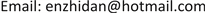Received: Nov. 22nd, 2018; accepted: Dec. 7th, 2018; published: Dec. 14th, 2018ABSTRACT

In this study, a finite element model is established based on the computational fluid dynamics. The velocity vector diagram and the streamline distribution of the different process parameters are obtained in the FSW process. The results show that with the increase of the rotating speed of the pin, the material flow near the pin is more violent and the effect of welding speed on material flow is not obvious. It is indicated from the simulation results that the welding speed can be enhanced to increase liquidity at the bottom of the weldment, but not to improve liquidity of the weldment surface.

Keywords:Computational Fluid Dynamics, Friction Stir Welding, Velocity Field, Flow Field1. 引言

2. CFD模型的建立

2.1. 控制方程

CFD数学模型将被焊材料假设为流体，流体是一种因受到剪切力作用而发生连续变形的物质，它具有连续性、易流动性、粘性和压缩性等物理性质，同时其流动时也满足质量守恒定律、动量守恒定律和能量守恒定律。CFD数学模型以这三个基本定律为基础，并以积分或者微分形式的方程组进行描述，把这些方程中的积分或者微分用离散的代数式来进行代替，把这些基本方程化为代数方程组；然后，通过用计算机来对这些代数方程组进行求解运算，就可以得出流动场在离散时间或者空间上的数值解    。

$\frac{\partial {u}_{i}}{\partial {x}_{i}}=0$ (1)

$\rho \frac{{u}_{j}\partial {u}_{i}}{\partial {x}_{j}}=-\frac{\partial P}{\partial {x}_{i}}+\frac{\partial }{\partial {x}_{j}}\left(\mu \frac{\partial {u}_{j}}{\partial {x}_{i}}+\mu \frac{\partial {u}_{i}}{\partial {x}_{j}}\right)$ (2)

$\stackrel{˙}{\epsilon }={\left(\frac{2}{3}{\epsilon }_{ij}{\epsilon }_{ij}\right)}^{1/2}$ (3)

${\epsilon }_{ij}$ 为应变率张量，其定义为：

${\epsilon }_{ij}=\frac{1}{2}\left(\frac{\partial {u}_{j}}{\partial {x}_{i}}+\frac{\partial {u}_{i}}{\partial {x}_{j}}\right)$ (4)

$\rho {C}_{p}\frac{{u}_{i}\text{d}T}{\text{d}{x}_{i}}=\frac{{u}_{i}\text{d}P}{\text{d}{x}_{i}}+{\nabla }^{2}kT-\frac{\partial {u}_{i}}{\partial {x}_{i}}P+\mu \left(\frac{\partial {u}_{i}}{\partial {x}_{j}}\cdot \frac{\partial {u}_{i}}{\partial {x}_{j}}\right)$ (5)

2.2. 材料模型

FSW稳态焊接过程中，焊缝金属的材料流动不仅受到焊接温度场的影响，还受到搅拌头形貌、焊接工艺和其与搅拌头接触状态等众多因素的影响。为建立能有效描述其流动行为的数学模型，必须对其进行抽象处理。并做简化与假设：假设焊缝材料为非牛顿流体。稳态焊接过程中，焊件温度并非恒定温度，而是呈一定规律分布。根据牛顿内摩擦定律可知，其流动速度梯度与剪应力大小不成正比，故其属于非牛顿流体；假设焊件流动材料为不可压缩流体。焊接过程中，焊缝材料流动的最大速度远小于搅拌头旋转线速度，表现为低速流动且材料本身不易被压缩，因此可将其视为不压缩流体。

${\sigma }_{e}=\sqrt{\frac{3}{2}\tau \cdot \tau }$ (6)

$\tau =2\mu \stackrel{˙}{\epsilon }$ (7)

${\sigma }_{e}=3\mu$ (8)

$Z=\stackrel{˙}{\epsilon }\mathrm{exp}\left(\frac{Q}{RT}\right)$ (9)

$\mu \left(T，\stackrel{˙}{\epsilon }\right)=\frac{1}{3\alpha }\mathrm{ln}\left\{{\left(\frac{Z\left(T，\stackrel{˙}{\epsilon }\right)}{A}\right)}^{\frac{1}{n}}+{\left[{\left(\frac{Z\left(T，\stackrel{˙}{\epsilon }\right)}{A}\right)}^{\frac{2}{n}}+1\right]}^{\frac{1}{2}}\right\}$ (10)Table 1. Material properties of 6061Al alloy used in model

2.3. 几何模型

3. 结果与分析

3.1. 速度场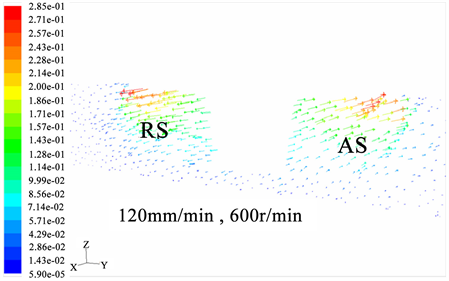(a)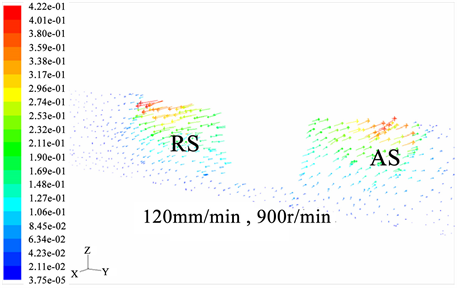(b)(c)

Figure 1. Vector diagram at different rotation velocities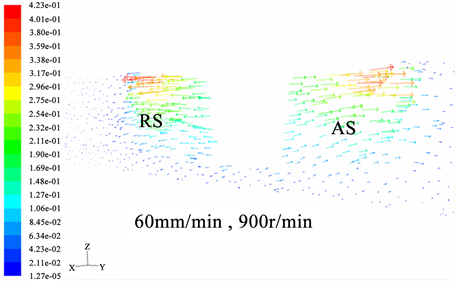(a)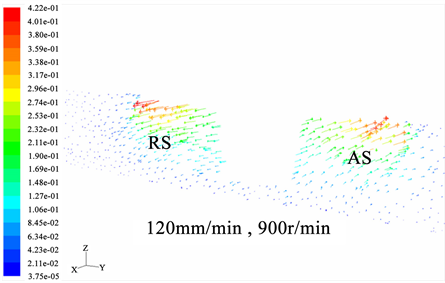(b)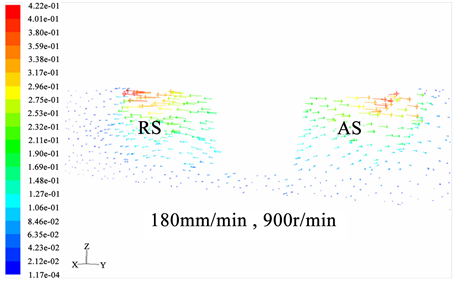(c)

Figure 2. Vector diagram at different welding velocities

3.2. 材料流动轨迹及应变率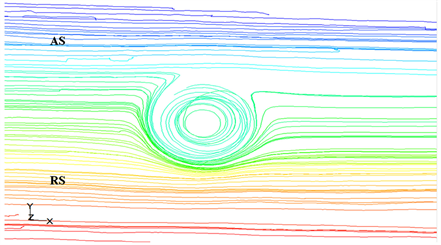Figure 3. Material streamline around pinFigure 4. Strain rate nephogram at different welding velocities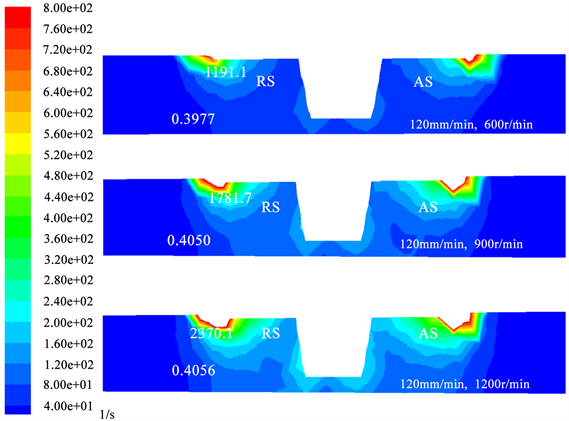Figure 5. Strain rate nephogram at different rotational velocities

4. 结论

1) 基于CFD理论建立lFSW过程有限元模型，模拟预测材料流动轨迹结果与实验相吻合，搅拌头及其附近区域的材料流动方向与搅拌头的旋转方向一致，AS和RS的材料均绕着搅拌头旋转，并在返回侧的相应位置释放。

2) 在FSW过程中，当搅拌头的旋转速度增加时，搅拌头附近区域材料流动更加剧烈；焊接速度的提高对搅拌头及其附近区域材料的流动影响不大。

Application of Computational Fluid Dynamics in Friction Stir Welding[J]. 流体动力学, 2018, 06(04): 134-142. https://doi.org/10.12677/IJFD.2018.64017

1. 1. Mishra, R.S. and Ma, Z.Y. (2005) Friction Stir Welding and Processing. Materials Science and Engineering: R: Reports, 50, 1-78. https://doi.org/10.1016/j.mser.2005.07.001

2. 2. Dialami, N., Cervera, M., Chiumenti, M. and de Saracibar, C.A. (2017) A Fast and Accurate Two-Stage Strategy to Evaluate the Effect of the Pin Tool Profile on Metal Flow, Torque and Forces in Friction Stir Welding. International Journal of Mechanical Sciences, 122, 215-227. https://doi.org/10.1016/j.ijmecsci.2016.12.016

3. 3. Pashazadeh, H., Teimournezhad, J. and Masoumi, A. (2014) Numerical Investigation on the Mechanical, Thermal, Metallurgical and Material Flow Characteristics in Friction Stir Welding of Copper Sheets with Experimental Verification. Materials and Design, 55, 619-632. https://doi.org/10.1016/j.matdes.2013.09.028

4. 4. Hasan, A.F., Bennett, C.J. and Shipway, P.H. (2015) A Numerical Comparison of the Flow Behaviour in Friction Stir Welding (FSW) Using Unworn and Worn Tool Geometries. Materials & Design, 87, 1037-1046. https://doi.org/10.1016/j.matdes.2015.08.016

5. 5. Wang, H., Colegrove, P.A. and dos Santos, J.F. (2013) Numerical Investiga-tion of the Tool Contact Condition during Friction Stir Welding of Aerospace Aluminium Alloy. Computational Materials Science, 71, 101-108. https://doi.org/10.1016/j.commatsci.2013.01.021

6. 6. Ulysse, P. (2002) Three-Dimensions Modeling of Friction Stir Welding Process. International Journal of Machine Tools & Manufacture, 42, 1549-1557. https://doi.org/10.1016/S0890-6955(02)00114-1

7. 7. Seidel, T.U. and Reynolds, A.P. (2003) Two-Dimensional Friction Stir Welding Process Model Based on Fluid Mechanics. Science and Technology of Welding and Joining, 8, 175-183. https://doi.org/10.1179/136217103225010952

8. 8. Zhang, Z. and Chen, J.T. (2012) Computational Investigations on Reliable Finite Element-Based Thermomechanical-Coupled Simulations of Friction Stir Welding. International Journal of Advanced Manu-facturing Technology, 60, 959-975. https://doi.org/10.1007/s00170-011-3651-5

9. 9. Albakri, A.N., Mansoor, B. and Nassar, H. (2013) Thermo-Mechanical and Metallurgical Aspects in Friction Stir Processing of AZ31 Mg Alloy—A Numerical and Experimental Investigation. Journal of Materials Processing Technology, 213, 279-290. https://doi.org/10.1016/j.jmatprotec.2012.09.015

10. 10. Schmidt, H. and Hattel, J. (2005) A Local Model for the Thermome-chanical Conditions in Friction Stir Welding Modelling. Modelling Simulation Materials Science Engineering, 13, 77-93. https://doi.org/10.1088/0965-0393/13/1/006